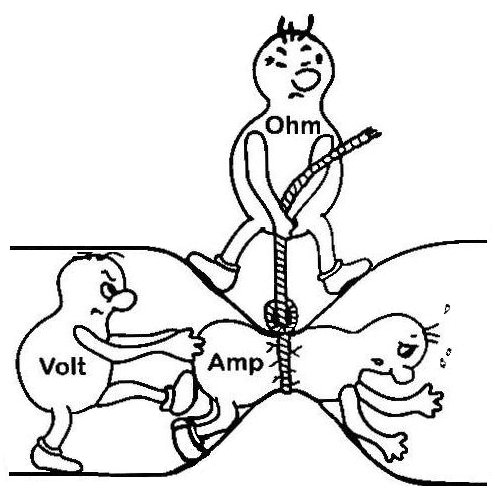## Kila, the Magic Husky's Ham Radio College

### featuring Kila and her Trusty Sidekick, BenEpisode 3 -- aired on February 29, 2016 -- Repeaters and Ohms, Amps, Volts and Watts; Ohm's Law; Resistance in series and parallel

Listen to the episode 57 MB MP3

• Callsign Regions
• How do we look up a callsign to find to whom it belongs? Ham Radio Callsigns contain a number which you can use to look up where they live.
• 2m Operations
• Half Duplex vs Full Duplex
• HT & Mobile Range
• Home Antennas, car antennas HT antennas
• Frequencies, band plan and # of channels. 144.00 - 147.99 every 15 Khz = 133
• The Band Plan allows only 70 pairs on 2 meters + 22 simplex FM
• 144.6 - 144.9 repeater inputs
• 145.2 - 145.5 repeater outputs (20 @15 KHz each)
• 146.01 - 146.37 repeater inputs
• 146.61 - 146.97 repeater outputs (24 @15 KHz each)
• 147.0 - 147.39 repeater outputs (26 @15 KHz each)
• 147.6 - 147.99 repeater inputs
• minus Satellite & weak signal work areas
• divided into Simplex and Duplex portions

• Repeaters
• Repeaters are early cell sites
• Most Ham Radios use "Half Duplex", a scheme wherein you may either talk or listen, but not both at the same time, as you can on a telelphone. To talk and listen at the same time requires "Full Duplex". In Half Duplex, you must press a "Push-to-talk" button, then wait up to half a second before you begin to speak, then talk, then release the button before you can hear anybody else. While you are talking, nobody else can hear anybody but you on that frequency. To get a good feeling for how this works, I recommend using the Zello app on your phone to talk to somebody.
• Squelch Tail and Courtesy Tone
• Offsets and CTCSS Tones and DCS signaling
• Repeaters are financed by Clubs
• Morning and Evening Commutes; Nets
• What is Cross-band Repeat and when might one use it?
• 2m IRLP and WinSystem

• DC Electronics; Volts, Amps, Ohms and Watts; Ohm's Law
• Volts, Ohms, Amperes and Watts
• Voltage = "Electromotive Force", E = Volts. One volt is defined as the electromotive force between two points required to cause one ampere of current to do one joule (measure of energy) of work in flowing between the points. Voltage can also be expressed as joules per coulomb of charge.
• Ohms = Resistance against the flow of current, in Ohms; R = Ohms
• Amperes = Current in Amps, or Current flow or amount of charge passing a point in a given time. I = Amps, 1 Ampere = 1 Coulomb per second. It is named after André-Marie Ampère (1775-1836), French mathematician and physicist, considered the father of electrodynamics. (Wikipeda)
• Watts = Power in Watts, P = Watts. 1 volt at 1 amp is 1 watt. 746 watts = 1 horsepower.

Regardless of how voltage is generated, energy must be supplied if current is drawn from the voltage source. The energy supplied may be in the form of chemical energy or mechanical energy. This energy is measured in joules (J). One joule is defined from classical physics as the amount of energy or work done when a force of one newton (a measure of force) is applied to an object that is moved one meter in the direction of the force.

Power is another important concept and measures the rate at which energy is generated or used. One watt (W) of power is defined as the generation (or use) of one joule of energy (or work) per second. One watt is also defined as one volt of EMF causing one ampere of current to flow through a resistance.
• Ohm's Law• E (Voltage in Volts) = I (Current in Amperes) × R (Resistance in Ohms)
• P (Power in Watts) = I (Current in Amperes) × E (Voltage in Volts)
• E = IR, therefore, R = E ÷ I and I = E ÷ R.
• P = IE, therefore, I = P ÷ E and E = P ÷ I.
• Wire resistance, that's why we need bigger wires for higher power or longer runs.
• Resistors in Series; R (total) = R(1) + R(2) + R(3) ...
• Resistor Color Code -- "Big Boys Race Our Young Girls But Violet Generally Wins" = "Black, Brown, Red, Orange, Yellow, Green, Blue, Violet, Gray, White".
• Resistor Tollerance colors (Red - 2%, Gold - 5%, Silver - 10%)
• Resistor types and power levels
• Voltage Drop
• Resistors in Parallel; R (total) = 1 ÷ ((1 ÷ R(1)) + (1 ÷ R(2)) + (1 ÷ R(3)) ...• Circuit Breakers and GFCI and AFCI circuit breakersIf I've made any mistakes, please Call me or email Kila or you can call us directly at (707) 413-6435.
Here is my main website.